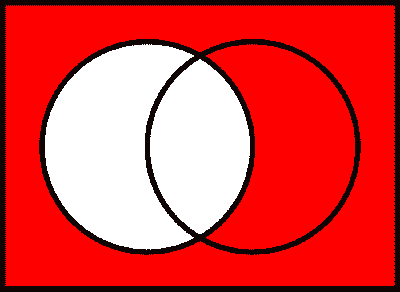# Set Theory Symbols

A comprehensive collection of the most notable symbols in set theory, categorized by function into tables along with each symbol's meaning and example.

Set theory is a branch of mathematics dedicated to the study of collections of objects, its properties, and the relationship between them. The following list documents some of the most notable symbols in set theory, along each symbol’s usage and meaning.

For readability purpose, these symbols are categorized by their function into tables. Other comprehensive lists of symbols — as categorized by subject and type — can be also found in the relevant pages below (or in the navigational panel).

Get the master summary of mathematical symbols in eBook form — along with each symbol’s usage and LaTeX code.

## Constants

In set theory, constants are often one-character symbols used to denote key mathematical sets. The following table documents the most notable of these — along with their respective meaning and example.

For other key sets of numbers, see key mathematical sets in algebra.

## Variables

Similar to other fields in mathematics, set theory often uses a designated list of variable symbols to refer to varying objects and quantities. The following table documents the most common of these — along with their respective example and meaning.

## Delimiters

In set theory, delimiters are symbols used to delineate the separation between independent mathematical entities, and often occur in the context of definition of sets. The following table documents the most common of these — along with their respective usage and meaning.

## Operators

Since the concept of set lies at the foundation of mathematics, many set operators — which allow one to refer to new sets through pre-existing sets — exist. The following table documents the most notable of these — along with their respective example and meaning.

The following diagrams, known as Venn diagrams, provide a visualization of five of the key sets mentioned above (where $A$ and $B$ are represented by the left and the right circle, respectively).$\overline{A}$

## Relational Symbols

In set theory, relational symbols are often used to describe relationships between sets, or relationships between a set and its element. The following table documents the most notable of these symbols — along with their respective meaning and example.

## Cardinality-related Symbols

In set theory, the concept of cardinality provides a way of quantifying and comparing the sizes of different sets. The following table documents some of the most notable symbols related to cardinality — along with each symbol’s usage and meaning.

(For a refresher on Greek and Hebrew symbols, see Greek, Hebrew and Latin-based symbols.)

For the master list of symbols, see mathematical symbols. For lists of symbols categorized by type and subject, refer to the relevant pages below for more.